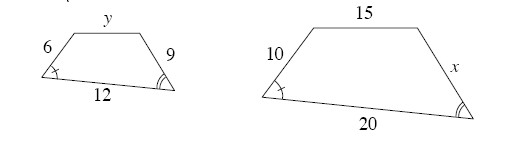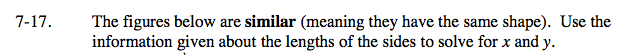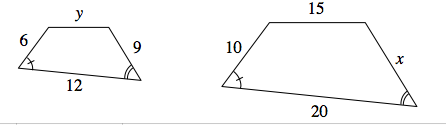### Home > AC > Chapter 7 > Lesson 7.1.2 > Problem7-17

7-17.

The figures below are similar (meaning they have the same shape). Use the information given about the lengths of the sides to solve for x and y. Homework Help ✎Use proportions to solve for x and y.

$\frac{6}{10}=\frac{\it y}{15}$

10y = 90

y = 9